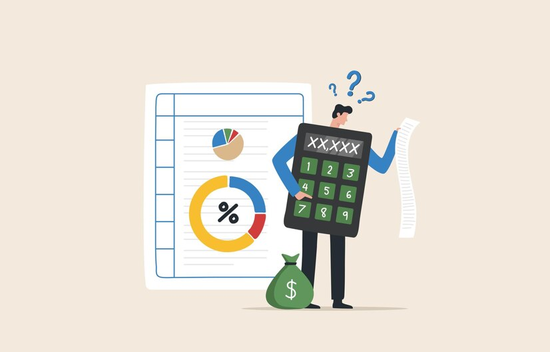A Comprehensive Guide on How to Calculate Marginal Cost in SingaporeAs a business owner or manager, understanding the concept of marginal cost can be crucial in making important decisions regarding pricing, production, and profitability. Marginal cost is the cost incurred in producing an additional unit of a product or service. In this article, we will discuss how to calculate marginal cost and how it can impact your business decisions.

To calculate marginal cost, you need to have a clear understanding of the various costs involved in producing a product or providing a service. These costs can be categorized into two types: fixed costs and variable costs.

Fixed costs are those that remain constant regardless of the level of production. Examples of fixed costs include rent, salaries, insurance, and equipment depreciation. Variable costs, on the other hand, increase or decrease depending on the level of production. Examples of variable costs include raw materials, direct labor, and utilities.

To calculate marginal cost, you need to first determine the variable cost per unit. This can be calculated by dividing the total variable cost by the total number of units produced. For example, if your total variable cost is \$10,000 and you have produced 1,000 units, then the variable cost per unit would be \$10.

Next, you need to calculate the change in total cost for producing one additional unit. This can be done by subtracting the total cost of producing the current number of units from the total cost of producing one more unit. For example, if the total cost of producing 1,000 units is \$15,000 and the total cost of producing 1,001 units is \$15,010, then the change in total cost for producing one additional unit would be \$10.

Finally, divide the change in total cost by the number of additional units produced. Using the example above, dividing \$10 by one additional unit would result in a marginal cost of \$10.

Understanding marginal cost can be helpful in making important business decisions such as setting prices or determining the level of production. If the marginal cost of producing an additional unit is less than the price at which the unit can be sold, then producing more units can increase profitability. On the other hand, if the marginal cost of producing an additional unit is more than the price at which the unit can be sold, then producing more units can decrease profitability.

In conclusion, understanding how to calculate marginal cost can provide valuable insights into your business operations and help you make informed decisions that can impact your bottom line. By taking into account both fixed and variable costs, you can gain a better understanding of the true cost of production and how it can impact your profitability.